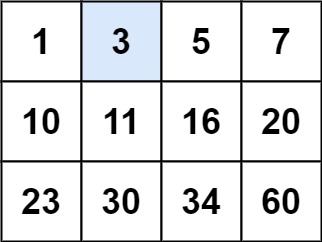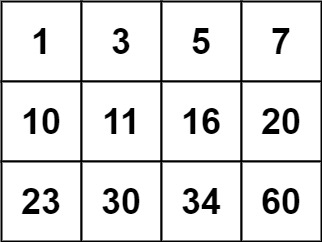# 74. Search a 2D Matrix | LeetCode

### 74. Search a 2D Matrix

Write an efficient algorithm that searches for a value in an `m x n` matrix. This matrix has the following properties:

• Integers in each row are sorted from left to right.
• The first integer of each row is greater than the last integer of the previous row.

Example 1:```Input: matrix = [[1,3,5,7],[10,11,16,20],[23,30,34,60]], target = 3
Output: true
```

Example 2:```Input: matrix = [[1,3,5,7],[10,11,16,20],[23,30,34,60]], target = 13
Output: false
```

Constraints:

• `m == matrix.length`
• `n == matrix[i].length`
• `1 <= m, n <= 100`
• `-104 <= matrix[i][j], target <= 104`

### The Solution to the above solution

class Solution {
public:
bool searchMatrix(vector<vector<int>>& matrixint target) {
int start=0;
int n=matrix.size();
int fir=matrix[start];
int last=matrix[start][n-1];
while(target>fir && target>last){
start++;
if(start>=matrix.size()){
return false;
}
fir=matrix[start];
last=matrix[start][n-1];
}
cout<<start<<endl;
int low=0;
int high=n-1,mid;
while(low<=high){
mid=(low+high)/2;
cout<<mid<<" ";
if(matrix[start][mid]==target){
return true;
}
else if(matrix[start][mid]<target){
low=mid+1;
}
else {
high=mid-1;
}
}
if(matrix[start][mid]==target){
return true;
}
return false;
}
};